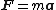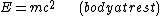# rest mass

Also found in: Dictionary, Thesaurus, Medical, Wikipedia.

## Rest mass

A constant intrinsic to a body which determines its inertial and energy-momentum properties. It is a fundamental concept of special relativity, and in particular it determines the internal energy content of a body. It is the same as the inertial mass of classical mechanics. According to the principle of equivalence, the basic physical principle of general relativity, the inertial mass of a body is also equal to its gravitational mass. See Classical mechanics, Gravitation, Relativity

The rest mass or inertial mass of a body, m, is a measure of its resistance to being accelerated at a by a force F ; in classical mechanics the relation between inertial mass, acceleration, and force is given by Newton's law, Eq. (1).

(1)In special relativity Newton's law holds exactly only in the body's rest frame, that is, the frame in which the body is instantaneously at rest. See Newton's laws of motion

Associated with the rest mass of a body, there is an internal or rest energy. In the system where the body is at rest, the energy of the body is given

(2)by Eq. (2).

The experimental realization of the interconversion of mass and energy is accomplished in the reactions of nuclei and elementary particles. In particular, the energy source of nuclear bombs and nuclear fission reactors is a small decrease in the total mass of the interacting nuclei, which gives rise to a large energy release because of the large numerical value of c2. See Elementary particle, Nuclear fission

McGraw-Hill Concise Encyclopedia of Physics. © 2002 by The McGraw-Hill Companies, Inc.

## rest mass

The mass of an elementary particle, etc., when at rest. According to the special theory of relativity, a body's mass increases as its speed increases. This is noticeable only at speeds close to the speed of light. Rest mass is often expressed in MeV (megaelectronvolts).
Collins Dictionary of Astronomy © Market House Books Ltd, 2006

## rest mass

[′rest ‚mas]
(relativity)
The mass of a particle in a Lorentz reference frame in which it is at rest.
McGraw-Hill Dictionary of Scientific & Technical Terms, 6E, Copyright © 2003 by The McGraw-Hill Companies, Inc.
References in periodicals archive ?
Rockenbauer shares that a multi-particle theory based on the strong and weak interaction can in all probability describe the quantum states where these properties mentioned are given as expectation values of the charge and rest mass operators.
"It is very often said in textbooks that the theory of relativity has shown that mass increases with velocity according to the relation m = [m.sub.0][gamma], where [m.sub.0] is the rest mass. Expressed in this way it appears as a new and profound property of matter, whereas it is really a result of a particular definition of relativistic velocity.
In  we applied this model on chain systems of harmonic quantum oscillators and could show that particle rest masses coincide with the eigenstates of the system.
PROPERTY ELECTRON rest mass m 9.10938356(11) x [10.sup.-31] kg energy E = [mc.sup.2] 0.5109989461(31) MeV angular frequency 7.76344071 x [10.sup.20] Hz [omega] = E/[??] angular oscillation period 1.28808867 x [10.sup.-21] s [tau] = 1/[omega] angular wavelength 3.8615926764(18) x [10.sup.-13] m [lambda] = c/[omega] angular acceleration 2.327421 x [10.sup.29] [ms.sup.-2] a = c[omega] PROPERTY PROTON rest mass m 1.672621898(21) x [10.sup.-27] kg energy E = [mc.sup.2] 938.2720813(58) MeV angular frequency 1.42548624 x [10.sup.24] Hz [omega] = E/[??] angular oscillation period 7.01515 x [10.sup.-25] s [tau] = 1/[omega] angular wavelength 2.1030891 x [10.sup.-16] m [lambda] = c/[omega] angular acceleration 4.2735 x [10.sup.32] [ms.sup.-2] a = c[omega]
If such a particle possessing the mass m is located at the point R = 0, then its rest mass density in the physical coordinates [[rho].sub.ph] = m[delta](R), and the potential of the produced gravitational field [phi] = -Gm/R is singular at the location point.
A neutrino with a magnetic moment, and one capable of changing from one kind to another, would also have to have a small rest mass. When the neutrino's existence was postulated more than 50 years ago, it was supposed to have zero rest mass.
PROPERTY ELECTRON rest mass m 9.10938356(11) x [10.sup.-31] kg energy E = [mc.sup.2] 0.5109989461(31) MeV angular frequency 7.76344071 x [10.sup.20] Hz [omega] = E/ft angular oscillation period 1.28808867 x [10.sup.-21] s [tau] = 1/[omega] angular wavelength 3.8615926764(18) x [10.sup.-13] m [lambda] = c/[omega] angular acceleration 2.327421 x [10.sup.29] [ms.sup.-2] a = c[omega] PROPERTY PROTON rest mass m 1.672621898(21) x [10.sup.-27] kg energy E = [mc.sup.2] 938.2720813(58) MeV angular frequency 1.42548624 x [10.sup.24] Hz [omega] = E/ft angular oscillation period 7.01515 x [10.sup.-25] s [tau] = 1/[omega] angular wavelength 2.1030891 x [10.sup.-16] m [lambda] = c/[omega] angular acceleration 4.2735 x [10.sup.32] [ms.sup.-2] a = c[omega]
That is, they constitute one possible kind of sterile neutrino, which may have to be invoked to fit simultaneously all the different 1998 measurements and hints of neutrino rest mass that are coming to us from laboratory experiments, observations of solar neutrinos, and anomalies in the numbers of neutrinos produced when cosmic rays hit the Earth's atmosphere.
A further question is whether neutrinos have a very small rest mass. Physicists have believed that neutrinos have exactly zero rest mass, but new theoretical developments would give them a tiny rest mass.
Therefore, the rest mass of the fundamental oscillator can be even smaller than the electron mass.
In order to change states, a neutrino must have at least a tiny amount of substance, or rest mass. However, neutrinos are assumed to be completely massless in the present theory uniting the electromagnetic force with the "weak" force that governs neutrino interactions with other particles.
Back around 1930, difficulties in balancingenergy in beta decay of atomic nuclei led Wolfgang Pauli to postulate the existence of a subatomic particle that had no rest mass, which Enrico Fermi named "little neutral one' or neutrino.

Site: Follow: Share:
Open / Close